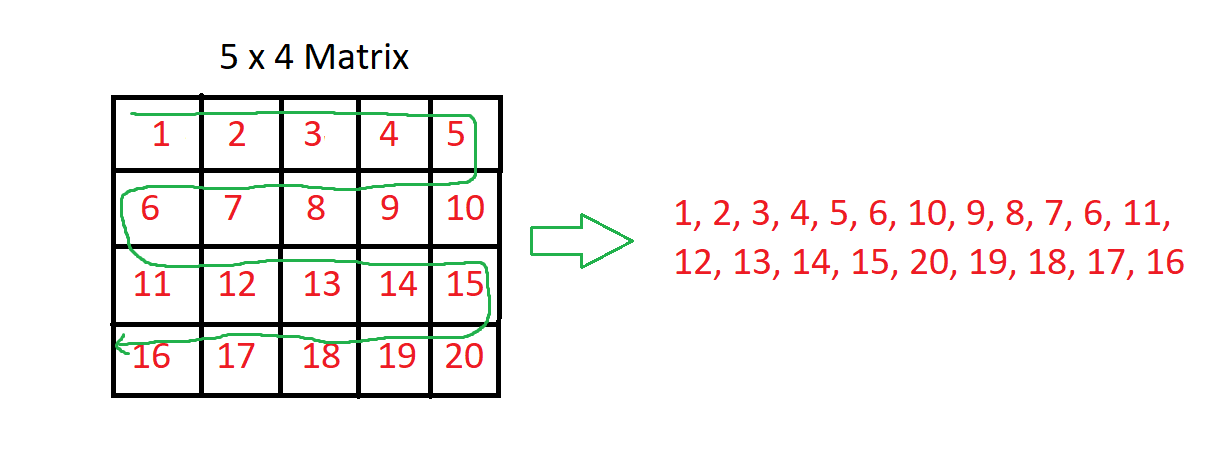# Matrix in Snake Pattern

Problem Statement: Given an N x N matrix. Your task is to print the elements of the matrix in the snake pattern.

Examples:

```Example 1:
Input: M = 4, N = 4 ,
array[M][N] = { {10, 20, 30, 40},
{15, 25, 35, 45},
{27, 29, 37, 48},
{32, 33, 39, 50}};
Output:
10, 20, 30, 40, 45, 35, 25, 15,
27, 29, 37, 48, 50, 39, 33, 32
Explanation: Printing the matrix in a snake pattern.

Example 2:
Input: M = 3, N = 3 , array[M][N] = { {1, 2, 3},
{4, 5, 6},
{7, 8, 9}};

Output: 1, 2, 3, 6, 5, 4, 7, 8, 9
Explanation: Printing the matrix in a snake pattern.```

### Solution

Disclaimer: Don’t jump directly to the solution, try it out yourself first.

### Solution: Using Nested For Loops

Approach:

Printing the snake pattern in the matrix is one of the basic problems.

The idea here is pretty simple, We will traverse all the rows.

For every row, we will check if it is even or odd. If the current row is even, we will print the current row from left to right i.e from 0 to N-1th index else we will print it from right to left i.e from N-1 to 0th index.Code:

## C++ Code

``````#include <bits/stdc++.h>

#define M 4 // number of rows
#define N 4 // number of columns
using namespace std;

void snakePrint(int mat[M][N]) {
// Traverse through all rows
cout << " Matrix in snake pattern : ";
for (int i = 0; i < M; i++) {

// If current row is even, print from
// left to right
if (i % 2 == 0) {
for (int j = 0; j < N; j++) {
cout << mat[i][j] << " ";
}

// If current row is odd, print from
// right to left
} else {
for (int j = N - 1; j >= 0; j--) {
cout << mat[i][j] << " ";
}
}
}
}

int main() {
// Declaring a Matrix of 4x4
int mat[M][N] = { { 10, 20, 30, 40 },
{ 15, 25, 35, 45 },
{ 27, 29, 37, 48 },
{ 32, 33, 39, 50 } };

snakePrint(mat); // Calling the snake print function
return 0;
}``````

Output: The matrix in Snake pattern is : 10 20 30 40 45 35 25 15 27 29 37 48 50 39 33 32

Time Complexity: O(N^2)

Reason: Traversing a matrix or a 2D array takes O(N^2) Because it has 2 nested loops.

Space Complexity: O(1)

## Java Code

``````import java.util.*;
class TUF {
static void print(int[][] mat) {
System.out.print("The matrix in Snake pattern is : ");
// Traverse through all rows
for (int i = 0; i < mat.length; i++) {

// If current row is even, print from
// left to right
if (i % 2 == 0) {
for (int j = 0; j < mat.length; j++)
System.out.print(mat[i][j] + " ");

// If current row is odd, print from
// right to left
} else {
for (int j = mat.length - 1; j >= 0; j--)
System.out.print(mat[i][j] + " ");
}
}
}

public static void main(String[] args) {
int mat[][] = new int[][]
{
{ 10, 20, 30, 40 },
{ 15, 25, 35, 45 },
{ 27, 29, 37, 48 },
{ 32, 33, 39, 50 }
};
print(mat);
}
}``````

Output: The matrix in Snake pattern is : 10 20 30 40 45 35 25 15 27 29 37 48 50 39 33 32

Time Complexity: O(N^2)

Reason: Traversing a matrix or a 2D array takes O(N^2) Because it has 2 nested loops.

Space Complexity: O(1)

Special thanks to Abhishek Yadav for contributing to this article on takeUforward. If you also wish to share your knowledge with the takeUforward fam, please check out this article